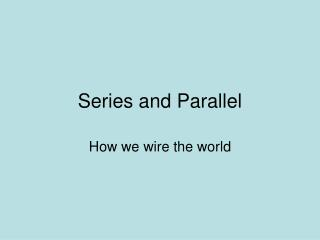Download PresentationSeries and Parallel

Series and Parallel - PowerPoint PPT Presentation

Series and Parallel. How we wire the world. Series vs Parallel Circuits. Parallel Circuit There are MULTIPLE paths for the current to flow through. Series Circuit Electrons only have one path to flow through. Series Circuit.I am the owner, or an agent authorized to act on behalf of the owner, of the copyrighted work described.
Download PresentationSeries and Parallel

Download Policy: Content on the Website is provided to you AS IS for your information and personal use and may not be sold / licensed / shared on other websites without getting consent from its author.While downloading, if for some reason you are not able to download a presentation, the publisher may have deleted the file from their server.

- - - - - - - - - - - - - - - - - - - - - - - - - - E N D - - - - - - - - - - - - - - - - - - - - - - - - - -
Presentation Transcript
1. Series and Parallel How we wire the world

2. Series vs Parallel Circuits • Parallel Circuit • There are MULTIPLE paths for the current to flow through. Series Circuit • Electrons only have one path to flow through.

3. Series Circuit • When electrons have to flow through one part to get to the next part • More components = more resistance • Increase resistance = decrease current (flow) • Less current = less bright bulbs • As voltage increases, current increases

4. Series Circuit – Pros & Cons Problems with Series: • The more devices (resistors) in a series circuit, the less current passes through (dimmer bulbs). • If one resistor breaks (a bulb goes out) the entire series is turned off.

5. Series Circuit - Resistance • Resistors – resists the flow of electrical current • Increased resistance will reduce the rate at which charge flows (aka current) • Total resistance goes UP with each resistor since the current has must go through each resistor. • Total Resistance = Sum of all resistors in the series Req = R1+R2+ R3…

6. Series Circuit - Current • Current = amount of charge (flow of electrons) • Like the flow of water • A current can't just disappear (appear) • Since only one path if some electrons flow through R1, then they have to continue flowing through R2 and R3. • Since the Current is the same through the entire circuit IT=I1=I2=I3

7. Series Circuit - Voltage • Voltage is the electric equivalent of water pressure. • The higher the voltage, the faster electrons will flow through the conductor. • Each component has resistance that causes a drop in voltage (reduction in voltage). • Total Voltage = The sum of voltages across each series resistors VT = V1 + V2 + V3…

8. Series vs Parallel Chart

9. Series Circuit - Example • Given • Vbattery = 12 V • R1 = 50 W, R2 = 100 W, R3 = 100 W • Complete the following table V = I R 1 2 3 ----------------------------------------------- T

10. Parallel Circuit – Pros and Cons Advantages • The more devices (resistors) in a parallel circuit, does not decrease the current (does not dim bulbs). • If one resistor breaks (a bulb goes out) the rest do not. Problems • Current doesn’t stay the same for entire circuit • So energy is used up quicker • So the total current increases = faster electrons = hotter wire = fire?

11. Which is better? Series or Parallel? Parallel • Most things are wired in parallel • Because of the fact that the more you plug in, the intensity doesn’t decrease. • Of course, this also increases the risk of fire • This is why homes have fuses or circuit breakers. They turn off everything in the circuit when current moves too fast.

12. Toll Booth Explanation • Adding toll booths in series increases resistance and slows the current flow. • Adding toll booths in parallel lowers resistance and increases the current flow.

13. Parallel Circuit - Resistance • Resistors added side-by-side • The more paths, the less TOTAL resistance. • 1/ Req=1/R1+1/R2+1/R3 • Ex. 2 resistors in parallel with 4Ω each. • Since the circuit offers two equal pathways for charge flow, only 1/2 the charge will choose to pass through a given branch.

14. Parallel Circuit - Current • ALL paths are used! • But the charge divides up into all branches • One branch can have more current than another branch (depends on resistance in branch). • Total current = sum of current in each path IT = I1 + I2 + …

15. Parallel Circuit - Voltage • A charge only passes through a single resistor. • Voltage drop across the resistor that it chooses to pass through must equal the voltage of the battery. • Total voltage = the voltage across each individual resistor • VT = V1 = V2 = …

16. Series vs Parallel Chart

17. Parallel Circuit - Example • Given • Vbattery = 12 V • R1 = 50 W, R2 = 100 W, R3 = 100 W • Complete the following table: V = I R 1 2 3 ----------------------------------------------- T

18. Two Types of Current • DC—Direct Current • produced by solar cells and chemical cells (batteries) • Current only flows in one direction. • AC—Alternating Current • Current flows back and forth (alternates) • Found in homes • Generators produce AC current